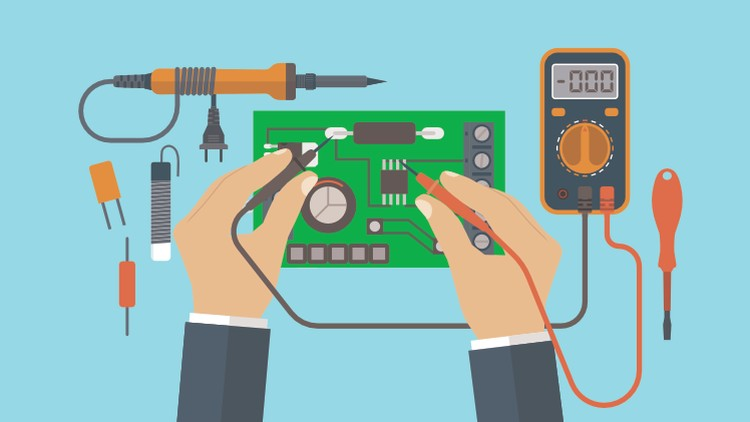### Basic Electronics [28-OCT-2019]

This course covers the theory of electrical and electronic principles. Students learn about the characteristics of electricity, the purpose and identification of electronic components, etc. It is designed to introduce to students, the basic concepts and principles of electronics.

Practical lab exercises involve the students constructing simple analog and digital circuits comparing measured values against values that are calculated. These lab sessions help to demonstrate the principles being taught.### Basic Electronics [03-SEP-2019]

This course covers the theory of electrical and electronic principles. Students learn about the characteristics of electricity, the purpose and identification of electronic components, etc. It is designed to introduce to students, the basic concepts and principles of electronics.

Practical lab exercises involve the students constructing simple analog and digital circuits comparing measured values against values that are calculated. These lab sessions help to demonstrate the principles being taught.